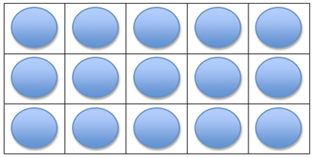Mathematics
Easy

Question

# Choose the multiplication expression that matches this array.## 2 × 5    3 × 5    5 × 5    3 × 3Hint:

## The correct answer is: 3 × 5

### In the question there is a array using which we have to find the multiplication expression of that array.Number of rows in the given array= 5Number of column in the given array= 3Multiplication expression of the given array= 3 × 5So, the multiplication expression of the given array is 3 × 5.Therefore, the correct option is b, i.e., 3 × 5.

For simple multiplication we have to remember the multiplication table of one-digit numbers and for two-digit number we can find it using the multiplication table of one-digit numbers. Here, we have to find the product of one digit number.

### Related Questions to study#### With Turito Foundation.#### Get an Expert Advice From Turito.